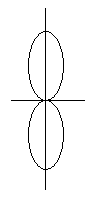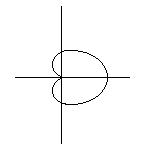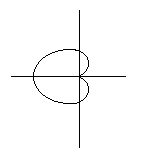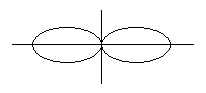# Electronics and Communication Engineering - Electromagnetic Field Theory

6.
A broadside array consisting of 200 cm wavelength with 10 half-wave dipole spacing 10 cm. And if each array element feeding with 1 amp. current and operating at same frequency then find the radiated power
730 W
73 W
100 W
None
Explanation:

Prad = n I2 . Rr10 x 73 x 1730 watt.

7.
For static magnetic field Maxwell's curl equation is given by
∇ .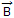= μ0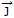∇ x= 0
∇ x= μ0∇ x= μ0/Explanation:

For static field, time varying factor will be zero, ∇ x H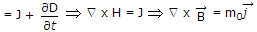.

8.
If wave of critical frequency 30 MHz is departing at an angle of 60°, then the MUF is given to be
10 MHz
15 MHz
30 MHz
60 MHz
Explanation:

MUF = fc sec θ

9.
An antenna having an effective temperature of 27°K is fed into a microwave amplifier that has an effective noise temperature of 30 K. Calculate the available noise power per unit bandwidth
30 K
57 K
27 K
3K, K is Boltzmann constant
Explanation: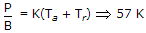where K is Boltzmann constant.

10.
Two identical and parallel dipole antenna are kept apart by a distance of λ/4 in the H-plane. They are fed with equal currents but the right most antenna has a phase shift of + 90°. The radiation pattern is given as